Question

An rectangular tank 5 m long, 2 m wide, and 8 m high is filled to a depth of 5 m of water. Set up the integral that would compute the work needed to pump the water out of a spout located at the top of the tank. Use the location of the origin and the direction of the positive axis as specified in the different parts. Note: Do not input values for the constant p9
(A) The origin is at the top of the tank with with positive y-values going down pg dy
(B) The origin is at the bottom of the tank with positive y-values going up. pg dy
(C) The origin is at the water level of the tank with positive y-values going down.dy

1.Giakhanh

Solution:

Given data:

A rectangular tank with dimensions 5 m as length, wide of 2 m and height of 8 m is filled with water tot a depth of 5 m.

A). If we select an elemental part of the tank of thickness dy at a depth y from the top, its mass will be :

m = p.v

⇒ m = p.( 5 x 2 dy)

⇒ m = 10 p dy

Now work done in moving mass from a depth y to the top will,

dw = m.g.y

dw = (10 p dy).g.y

or dw = 10 p.g.y.dy

Total work done is :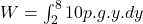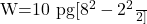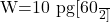W = 300 p.g

B). Origin at the bottom and consider an elemental part of the tank at a height y from the bottom, then its mass is :

⇒ m = p.( 5 x 2 dy)

⇒ m = 10 p dy

Now, work done in moving this mass from a height y above origin to a height 8 m above the origin is,

dw = mg(8 – y)

dw = (10 p dy).g.(8 – y)

dw = 10 pg.(8 – y). dy

The total work done is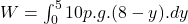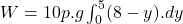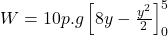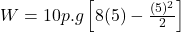W = 10 pg (40-12.5)

W = 275 pg

C). Origin at the water level and consider y axis positive in the downward direction.

The work done to move and elemental ,as from a depth y below the water level to the top of the tank is,

dw = 10 pg (y+2) dy

The total work done is :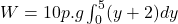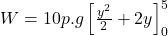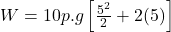W = 10 p g (12.5 + 10)

W = 225 pg